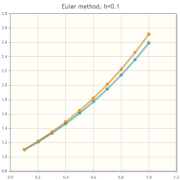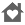Runge-Kutta MethodsEveryone
2
Runge-Kutta Methods is a powerful application to help solving in numerical intitial value problems for differential equations and differential equations systems.

Runge-Kutta Methods can solve initial value problems in Ordinary Differential Equations systems up to order 6.

Also, Runge-Kutta Methods, calculates the An , Bn coefficients for Fourier Series representation.

You can select over 12 integration methods including Runge-Kutta including Fehlberg and Dormand and Prince methods. From most simply Euler Method (order 1) to New65 (order 6).

Program opens with a default initial value problem:

y' = y ,
y(0)= 1

For integration interval: [0, 1]
wich admits the inmediate and obvous solution y=exp(x).

*********************** INPUTS *************************

Select Simple ODE Solver mode if your problem has only an equation or System ODEs mode if you has a System or Ordinary equations.

1) Enter the initial value for the independent variable, x0.
2) Enter the final value for the independent variable, xn.
3) Enter the step size for the method, h.
4) Enter the given initial value of the independent variable y0.
5) Select from the combo the integration method (default Euler method is selected).
6) Enter the function f(x, y) of your problem, for example.

if f(x,y) = e2xy -> enter e^(2*x*y)
if f(x,y) = sin e2xy -> enter sin(e^(2*x*y))

6) Enter exact solution if known for the estimation of statistical Runge-Kutta methods error.

********************** NOTE THAT ***********************

The calculations using numerical methods are subject to two kind of errors

1)Truncation mehod error

2)Error due to limitation of computer arithmetic

This app requires interner connection!!

Enjoy it!!
Collapse

Review Policy
2.0
2 total
5
4
3
2
1

What's New

- Accuracy selection
- Off line Runge-kutta method execution
Collapse

Eligible for Family LibraryLearn More
Updated
January 13, 2017
Size
7.8M
Installs
100+
Current Version
5.2
Requires Android
2.2 and up
Content Rating
Everyone
Permissions
Offered By
Carlos Mathstools
Developer
calle jacinto gomez comesaña, 17 madrid 28038